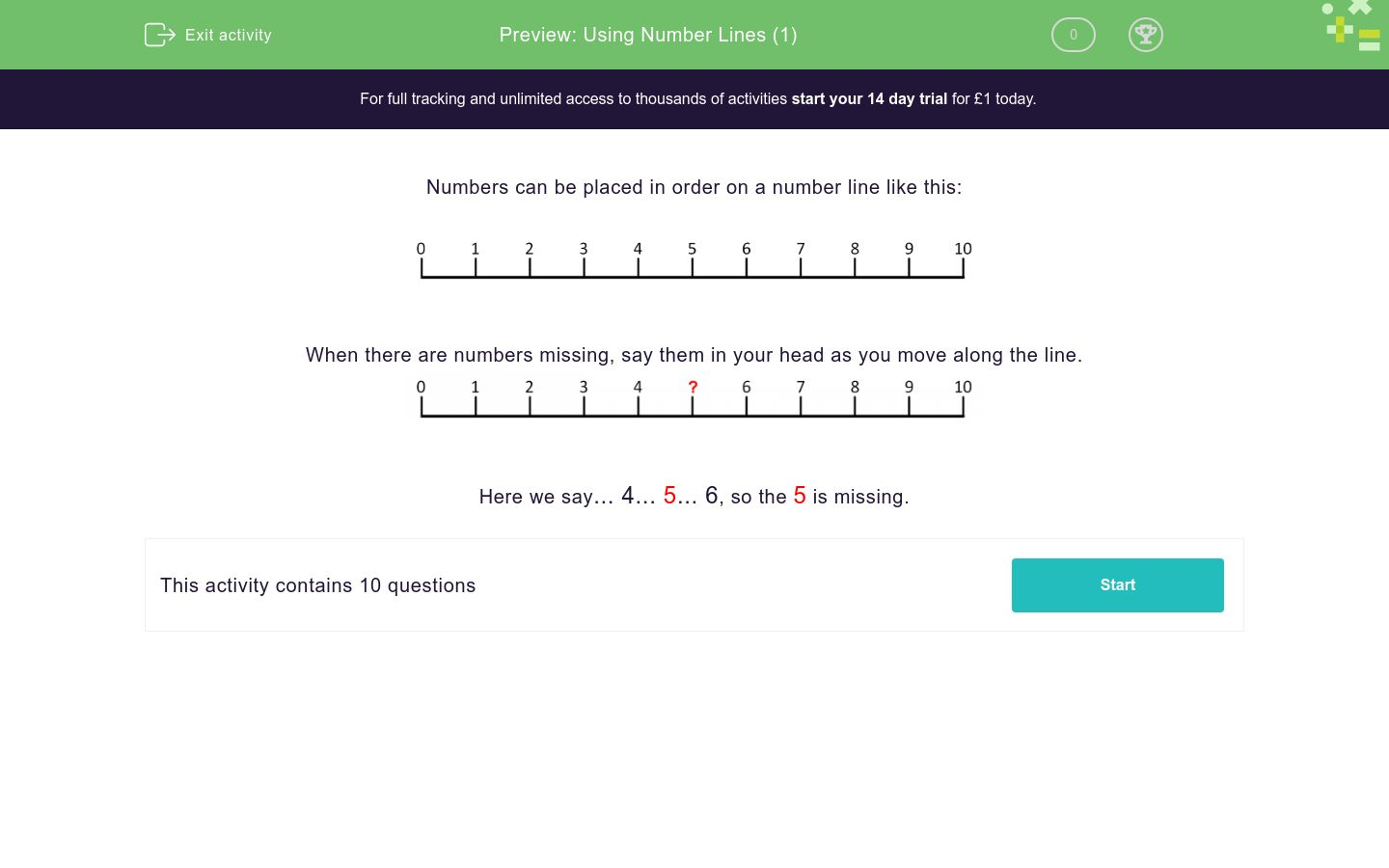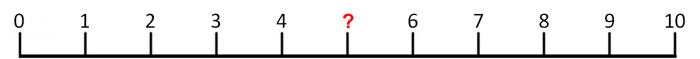# Using Number Lines (1)

In this worksheet, students must identify the missing number on a number line.Key stage:  KS 1

Curriculum topic:   Number: Number and Place Value

Curriculum subtopic:   Represent Numbers to 100

Difficulty level:### QUESTION 1 of 10

Numbers can be placed in order on a number line like this:When there are numbers missing, say them in your head as you move along the line.Here we say... 4... 5... 6, so the 5 is missing.

What is the missing number on this number line?What is the missing number on this number line?What is the missing number on this number line?What is the missing number on this number line?What is the missing number on this number line?What is the missing number on this number line?What is the missing number on this number line?What is the missing number on this number line?What is the missing number on this number line?What is the missing number on this number line?• Question 1

What is the missing number on this number line?2
EDDIE SAYS
2 comes between 1 and 3.
• Question 2

What is the missing number on this number line?5
EDDIE SAYS
5 comes between 4 and 6.
• Question 3

What is the missing number on this number line?6
EDDIE SAYS
6 comes between 5 and 7.
• Question 4

What is the missing number on this number line?1
EDDIE SAYS
1 comes between 0 and 2.
• Question 5

What is the missing number on this number line?0
EDDIE SAYS
0 is right at the start and comes before 1.
• Question 6

What is the missing number on this number line?4
EDDIE SAYS
4 comes between 3 and 5.
• Question 7

What is the missing number on this number line?8
EDDIE SAYS
8 comes between 7 and 9.
• Question 8

What is the missing number on this number line?7
EDDIE SAYS
7 comes between 6 and 8.
• Question 9

What is the missing number on this number line?9
EDDIE SAYS
9 comes between 8 and 10.
• Question 10

What is the missing number on this number line?10
EDDIE SAYS
10 is at the end after 9.
---- OR ----

Sign up for a £1 trial so you can track and measure your child's progress on this activity.

### What is EdPlace?

We're your National Curriculum aligned online education content provider helping each child succeed in English, maths and science from year 1 to GCSE. With an EdPlace account you’ll be able to track and measure progress, helping each child achieve their best. We build confidence and attainment by personalising each child’s learning at a level that suits them.

Get started